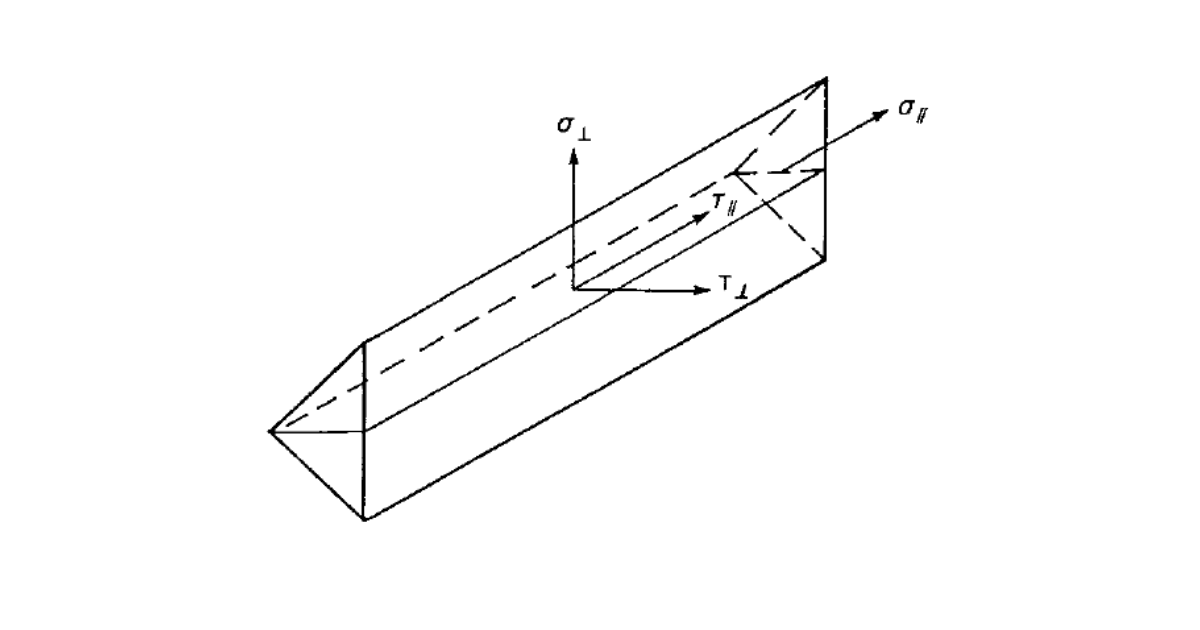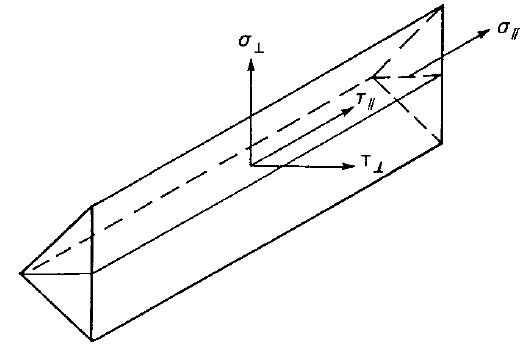### Choose language# Code-check of welds according to Hong Kong Code

$$Butt weldsFull penetration butt welds are expected and their resistance is considered equal to that of the parent metal – Cl. 9.2.5.2.1.Fillet weldsFillet welds are designed by Simplified method according to Cl. 9.2.5.1.6. $f_w \le p_w$ $$f_w = \sqrt{\sigma_\perp ^2 + \tau_\perp ^2 + \tau_\parallel ^2}$$ – vector sum of stresses in weld throat in all directions $$p_w$$ – design strength of fillet weld determined according to Table 9.2a and 9.2b; for cases not covered by Tables 9.2a and 9.2b: $$p_w = \min \{0.5 U_e, 0.55 U_s\}$$ – for EN electrode used with EN steel $$p_w = 0.38 \min \{U_e, U_s\}$$ – for other cases $$U_e$$ – minimum tensile strength of electrode $$U_s$$ – minimum tensile strengthThe effective length of the fillet weld is reduced by $$2\cdot s$$ according to Cl. 9.2.5.1.3, where $$s$$ is the fillet weld leg size assumed equal to $$a\cdot \sqrt{2}$$. Electrode Steel grade354250 S 275220220220 S 355220250250 S 460220250280 Steel gradeElectrodeDesign strength Q235E43160 Q345E50200 Q390, Q420E55220 Tables 9.2a and 9.2b: Design strengths $$p_w$$ [MPa] Welding electrodeMinimum tensile strength $$U_e$$ [MPa] 35440 42500 50560 E43421.1 E50526.3 E55578.9 Default minimal tensile strength of electrode $$U_e$$ [MPa]The weld diagrams show stress according to the following formula:$\sigma = \sqrt{\sigma_{\perp}^2 + \tau_{\perp}^2 + 3 \tau_{\parallel}^2 }$$$